# Solutions of Electricity (Page No- 19) - Physics By Lakhmir Singh, Class 10 Class 10 Notes | EduRev

## Class 10 : Solutions of Electricity (Page No- 19) - Physics By Lakhmir Singh, Class 10 Class 10 Notes | EduRev

The document Solutions of Electricity (Page No- 19) - Physics By Lakhmir Singh, Class 10 Class 10 Notes | EduRev is a part of the Class 10 Course Class 10 Physics Solutions By Lakhmir Singh & Manjit Kaur.
All you need of Class 10 at this link: Class 10

Lakhmir Singh Physics Class 10 Solutions Page No:19

Question 15:
What is Ohm’s law ? Explain how it is used to define the unit of resistance.
Solution :
Ohm’s law gives a relationship between current (I) and potential difference (V). According to ohm’s law: At constant temperature, the current flowing through a conductor is directly proportional to the potential difference across its ends.
If I is the current flowing through a conductor and V is the p.d. across its ends, then according to the ohm’s law:

where, R is a constant called "resistance" of the conductor. The unit of resistance is ohm.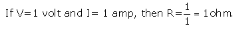Thus, 1 ohm is  the resistance of a conductor such that when a potential differenoe ot 1 volt is applied to its ends, a current of 1 amp flows through it.

Question 16:
(a) What is meant by the “resistance of a conductor” ? Write the relation between resistance, potential
difference and current.
(b) When a 12 V battery is connected across an unknown resistor, there is a current of 2.5 mA in the circuit. Calculate the value of the resistance of the resistor.

Solution :
(a) The property of a conductor due to which it opposes the flow of current through it is called resistance of the conductor.
Work done = Potential difference x charge moved.
(b) V = 12volt, I=2.5 x 10-3 A
We know that
V=IR
R=V/I
R=12/(2.5X10-3)
R=4.8X103ohm = 4800 ohm.

Question 17:
(a) Define the unit of resistance (or Define the unit “ohm”).
What happens to the resistance as the conductor is made thinner ?
Keeping the potential difference constant, the resistance of a circuit is doubled. By how much does the current change ?

Solution :
(a) 1 ohm is the resistance of a conductor such that when a potential difference of 1 volt is applied to its ends, a current of 1ampere flows through it.
(b) Its resistance will increase.
(c)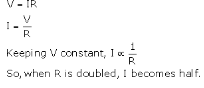Question 18:
(a) Why do electricians wear rubber hand gloves while working with electricity ?
(b) What p.d. is needed to send a current of 6 A through an electrical appliance having a resistance of 40 Ω ?

Solution :
(a) Electricians wear rubber hand gloves while working with electricity because rubber is an insulator and protects them from electric shocks.
(b) I=6amp, R=40ohm
We know that
V=IR
V = 6 x 40 = 240 V.

Question 19:
An electric circuit consisting of a 0.5 m long nichrome wire XY, an ammeter, a voltmeter, four cells of 1.5 V each and a plug key was set up.
(i) Draw a diagram of this electric circuit to study the relation between the potential difference maintained between the points ‘X’ and ‘Y’ and the electric current flowing through XY.
(ii) Following graph was plotted between V and I values :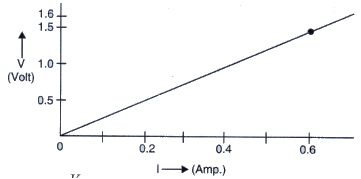(iii) What is the resistance of the wire ?
Solution :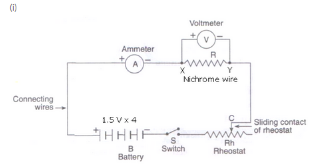(ii) Since the graph is a straight line passing through the origin so current is directly proportional to the potential difference. Hence, die ratior V/I remains constant.

From graoh, when V = 1.5 volt 1 = 0.6 amp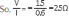For p.d 0.8V, 1.2v and 1.6V. the value of V/I ratio remains the same i.e., 2.5 ohm

The resistance of the wire is. equal to the ratio of potential difference applied and the currrent passing through it.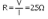Question 20:
(a) What is the ratio of potential difference and current known as ?
(b) The values of potential difference V applied across a resistor and the corresponding values of current I
flowing in the resistor are given below :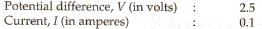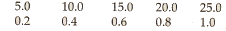Plot a graph between V and I, and calculate the resistance of the resistor.

(c) Name the law which is illustrated by the above V-I graph.
(d) Write down the formula which states the relation between potential difference, current and resistance,
(e) The potential difference between the terminals of an electric iron is 240 V and the current is 5.0 A. What is the resistance of the electric iron ?

Solution :
(a) The ratio of potential difference and current is known as resistance.
(c) Ohm’s law
(d) Potential difference = Current x Resistane
(e) V = 240 volt, I = 5amp
We know that
V = IR
240 = 5 x R
R = 240/5 = 48 ohm.
PAGE 30:

Question 30:
An electric room heater draws a current of 2.4 A from the 120 V supply line. What current will this room heater draw when connected to 240 V supply line ?
Solution :
In first case,
I = 2.4 amp, V = 120 volt
V=IR
120 = 2.4 x R
R = 120/2.4 = 50 ohm
In second case,
V = 240 volt, R = 50 ohm
V = IR
240 = I x 50
I = 4.8 amp.

Question 31:
Name the electrical property of a material whose symbol is “omega”.
Solution :
Resistance.

Question 32:
The graph between V and 1 for a conductor is a straight line passing through the origin.
Which law is illustrated by such a graph ?
What should remain constant in a statement of this law ?

Solution :
(a) Ohm’s law
(b) Temperature.

Question 33:
A p.d. of 10 V is needed to make a current of 0.02 A flow through a wire. What p.d. is needed to make a current of 250 mA flow through the same wire ?
Solution :
In first case,
I = 0.02 amp, V = 10 volt
V = IR
10 = 0.02 x R
R = 10/0.02 = 500 ohm
In second case,
I = 250 x 10-3 amp, R = 500 ohm
V = IR
V = 250 x 10-3 x 500
V = 125 volt.

Question 34:
A current of 200 mA flows through a 4 kΩ resistor. What is the p.d. across the resistor ?
Solution :
I = 200mA = 0.2 A
R = 4 x 103ohm = 4000 ohm
We know that
V = IR
V = 0.2 x 4000
V = 800 volt.

Offer running on EduRev: Apply code STAYHOME200 to get INR 200 off on our premium plan EduRev Infinity!

94 docs

,

,

,

,

,

,

,

,

,

,

,

,

,

,

,

,

,

,

,

,

,

,

,

,

;